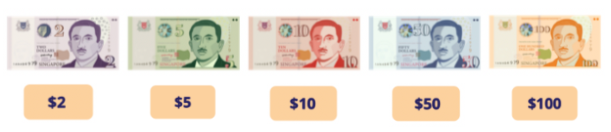Study P2 Mathematics Money 1 - Geniebook# Money 1

1. Writing amount of money in figures
2. Writing amount of money in words
3. Count amount of money
4. Comparing amounts of money

## 1. Writing Amount Of Money In Figures

We use ‘$’ to represent dollars and ‘¢’ to represent cents. Example 1: One dollar $$= 1$$ Ten dollars $$= 10$$ Fifty cents $$= 50¢$$ Note: ¢ will be written after the amount ) However, if we were to write dollars and cents together, we will use the sign ‘$’ and a decimal point ‘.’ instead.

Example 2:

One dollar and fifty cents $$= 1.50$$

Twelve dollars and thirty-five cents $$= 12.35$$

Question 1:

Write the following amount of money in figures:

Fifteen dollars and twenty cents

1. $15.02 2.$15.20
3. $50.02 4.$50.20

(2) $15.20 Question 2: Write the following amount of money in figures: Twenty dollars and twenty cents 1.$20.02
2. $20.20 3.$22.02
4. $22.22 Answer: (2)$20.20

## 2. Writing Amount Of Money In Words

When writing the amount of money in words, the decimal point is used to separate the dollars and cents. The numbers on the left of the ‘.’ represent the amount in dollars and the numbers on the right of the ‘.’ represent the amount in cents.

Example 1:

$$0.80 =$$ Eighty cents

$$15.30 =$$ Fifteen dollars and thirty cents

$$100 =$$ One hundred dollars

$$25 =$$ Twenty-five dollars

Question 1:

Write the following amount of money in words:

$24.35 1. Two four dollars and three five cents 2. Twelve dollars and thirty cents 3. Twenty dollars and thirty-five cents 4. Twenty-four dollars and thirty-five cents Answer: (4) Twenty-four dollars and thirty-five cents Question 2: Write the following amount of money in words:$256

1. Two dollars and fivety-six cents
2. Two hundred dollars and fifty-six cents
3. Two hundred and fifty-six dollars
4. Two hundred and fifty-six cents

(3) Two hundred and fifty-six dollars

## 3. Count Amount Of Money

To count the amount of money, we will first need to identify the value of each note and coin. From there, we will be able to add the value of each note and/or coin to determine the total amount of money.

Identifying the value of coins in Singapore:Identifying the value of notes in Singapore:Example 1:

Count and write the amount of money.Solution:

Three $1-coins $$= 3$$ Two 50¢-coins $$= 1$$ Two 50¢-coins $$= 1$$ Three 10¢-coins $$= 30¢$$ Two 5¢-coins $$= 10¢$$ Answer:$5.40

Question 1:

What is the total value of these coins?1.  $1.20 2.$1.80
3.  $5.20 4.$5.40

(2) $1.80 Question 2: What is the total value of these coins?1.$2.15
2.  $2.55 3.$2.65
4.  $5.15 Answer: (3)$2.65

Question 3:

Count and write the total amount of money.1. $4.50 2.$5.00
3. $8.50 4.$9.00

(4) $9.00 Question 4: Count and write the total amount of money.1.$5.60
2. $14.60 3.$17. 60
4. $19.60 Answer: (4)$19.60

## 4. Comparing Amounts Of Money

To compare the amount of money, we need to first compare the numbers representing the amount in dollars, followed by the numbers representing the amount in cents.

In other words, we will compare the digits from left to right.

• $15.50 costs more than$11.50 as 15 is greater than 11.
• Between $11.50 and$11.10, since $11 is common, we will compare the digits after the decimal, which is the cents.$11.50 costs more than $11.10 as 50 is greater than 10. Example 1: Which one of the following is the smallest amount of money? 1.$12.35
2. $12.55 3.$13.55
4. $15.25 Solution:$12.35 and $12.55 cost less than$13.55 and $15.25, as 12 is smaller than 13 and 15. Comparing the cents in Options (1) and (2), 35¢ costs lesser than 55¢ since 35 is smaller than 55. So, Option (1) is the smallest amount of money. Answer: (1)$12.35

Question 1:

Which one of the following is the smallest amount of money?

1. $13.10 2.$13.30
3. $30.10 4.$30.30

(1) $13.10 Question 2: Which of the following is the greatest amount of money? 1.$19.05
2. $19.15 3.$19.50
4. $19.55 Answer: (4)$19.55

Question 3:

Which of the following bags is the most expensive?1. Bag A
2. Bag B
3. Bag C
4. Bag D

(4) Bag D

Question 4:

Who has more savings in her piggy bank?1. Amanda
2. Belinda

Solution:

Amanda has 80¢ in her piggy bank.

Belinda has 70¢ in her piggy bank.

So, Amanda has more savings.

(1) Amanda

Question 5:

Alice has three $2 notes and three$1 coins.

Belle has three $2 notes and three 50¢ coins. Cindy has two$5 notes and two 5¢ coins.

Who has the most amount of money?1. Alice
2. Belle
3. Cindy

Solution:

Alice has $9, Belle has$10.50 and Cindy has \$10.10.

So, Belle has the most amount of money.

(2) Belle

• Writing amount of money in figures
• Writing amount of money in words
• Count the amount of money
• Comparing amounts of money

Continue Learning
Numbers To 1000 Multiplication And Division 1
Multiplication And Division 2 Addition And Subtraction 1
Addition And Subtraction 2 Fractions 1
Length 1 Mass 1
Volume 1 Money 1
Time 1 Shapes And Patterns
Picture Graphs 1 Model Drawing 1
Model Drawing 4PrimaryPrimary 1Primary 2EnglishMaths
Numbers To 1000
Multiplication And Division 1
Multiplication And Division 2
Fractions 1
Length 1
Mass 1
Volume 1
Money 1
Time 1
Shapes And Patterns
Picture Graphs 1
Model Drawing 1
Model Drawing 4Primary 3Primary 4Primary 5Primary 6SecondarySecondary 1Secondary 2Secondary 3Secondary 4